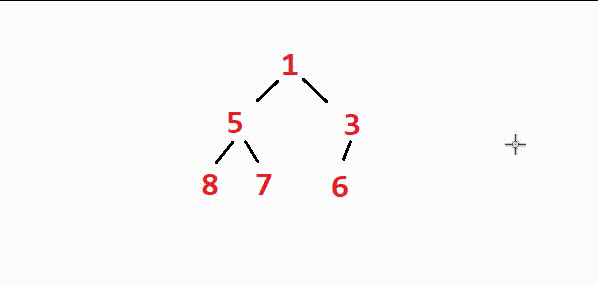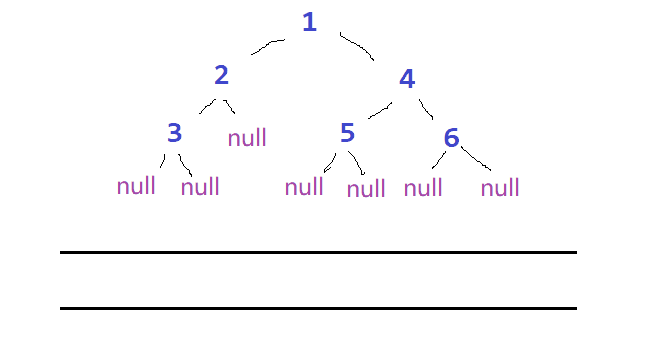# 1 二叉树的概念及结构

## 1.1 二叉树的概念

1. 为空
2. 由一个根结点和两棵分别名为左子树和右子树的二叉树组成1. 二叉树中没有度大于2的结点
2. 二叉树的子树有左右之分，次序不能颠倒，因此二叉树是有序树## 1.2 特殊的二叉树

1. 满二叉树： 一个二叉树，如果每一个层的结点数都达到最大值，则这个二叉树就是满二叉树。也就是 说，如果一个二叉树的层数为K，且结点总数是 ，则它就是满二叉树。
2. 完全二叉树： 完全二叉树是效率很高的数据结构，完全二叉树是由满二叉树而引出来的。对于深度为K 的，有n个结点的二叉树，当且仅当其每一个结点都与深度为K的满二叉树中编号从1至n的结点一一对 应时称之为完全二叉树。 通俗地理解，完全二叉树最后一层的结点必须是从左到右连续的，其他层的结点都是满的。 要注意的是满二叉树是一种特殊的完全二叉树。

`满二叉树一定是完全二叉树，二叉树不一定是满二叉树。`## 1.3 二叉树的性质

1. 若规定根节点的层数为1，则一棵非空二叉树的第i层上最多有 2i-1 个结点
2. 若规定根节点的层数为1，则深度为h的二叉树的最大结点数是 2h-1 （计算方法：20 +21+22+…+2h-1 = 2h-1）
3. 对任何一棵二叉树,，如果度为0的叶结点个数为n0，度为2的分支结点个数为n2，则有 n0 = n2 + 1
4. 完全二叉树中，假设总结点数为n，度为1的结点数为n1，则 当n为偶数时，n1 = 1，n为奇数时，n1 = 0
5. 若规定根节点的层数为1，具有n个结点的满二叉树的深度， h = log(n+1) （ps：log(n+1)是log以2为底，n+1为对数）

6. 若一颗二叉树的结点个数为n,则： n = n0+n1+n2，n-1 = 0*n0 + 1*n1 + 2*n2
7. 对于具有n个结点的完全二叉树，如果按照从上至下从左至右的数组顺序对所有节点从0开始编号，则对于序号为i的结点有：

1️⃣某二叉树共有 399 个结点，其中有 199 个度为 2 的结点，则该二叉树中的叶子结点数为（ ）
A 不存在这样的二叉树
B 200
C 198
D 1992️⃣在具有 2n 个结点的完全二叉树中，叶子结点个数为（ ）
A n
B n+1
C n-1
D n/23️⃣一棵完全二叉树的节点数位为531个，那么这棵树的高度为（ ）
A 11
B 10
C 8
D 12## 1.4 二叉树的存储结构

1. 顺序结构2. 链式结构``````typedef int DataType;
struct BinaryTreeNode
{

struct BinaryTreeNode* leftChild;//指向当前结点左孩子
struct BinaryTreeNode* rightChild;//指向当前结点右孩子
DataType val;//当前结点数据域
};
``````

# 2 二叉树的顺序结构及实现

## 2.2 什么是堆？

• 堆总是一颗完全二叉树
• 堆中某个节点的值总是不大于或不小于其父节点的值

1. 小根堆2. 大根堆## 2.3 堆的实现

### 2.3.1 堆的向下、向上调整算法

#### 1️⃣向下调整算法

``````int a[] = {
27,15,19,18,28,34,65,49,25,37};
```````不仅是根节点可以进行向下调整，其他任何满足左右子树都是一个堆的节点都可以进行向下调整。`

``````void Swap(int& a, int& b)
{

int tmp = a;
a = b;
b = tmp;
}
// 向下调整算法
void AdjustDown(HeapDataType* a, int n, int parent)
{

assert(a);
int TheChild = parent * 2 + 1;
//
while (TheChild < n)//孩子的范围必须在数组下标范围内
{

//找到最小(最大)孩子
if (TheChild + 1 < n && a[TheChild + 1] < a[TheChild])//根据建立大小堆改变符号
{

TheChild++;
}
//判断：最小(最大)孩子和父亲是否交换
if (a[TheChild] < a[parent])
{

Swap(a[parent], a[TheChild]);
//移动父亲和孩子的下标
parent = TheChild;
TheChild = parent * 2 + 1;
}
else//不用交换，则终止调整，跳出循环
{

break;
}
}
}
``````

#### 1️⃣向上调整算法(堆的插入)

``````int a[] = {
15,18,19,25,28,34,65,49,27,37 };
``````1. 先将元素插到堆的末尾，既最后一个元素后面
2. 向上调整，恢复堆结构

``````void AdjustUp(int* a, int child)
{

assert(a);
int parent = (child - 1) / 2;
while (child > 0)
{

if (a[child] < a[parent])
{

Swap(a[child], a[parent]);
child = parent;
parent = (child - 1) / 2;
}
else
{

break;
}
}
}
``````

### 2.3.2 堆的创建

``````int a[] = {
1,5,3,8,7,6};
````````````//a {1,5,3,8,7,6}
void HeapCreat(HeapDataType* a, int n)
{

assert(a);
//找到第一个调整的节点
int aN = ((n - 1) - 1) / 2;
//第一个调整的节点往前的每个节点都要向下调整
while (aN >= 0)
{

--aN;
}
}
``````### 2.3.3 堆创建的时间复杂度### 2.3.4 堆的删除### 2.3.5 堆的总体代码实现

Heap.h

``````#include <stdio.h>
#include <stdlib.h>
#include <assert.h>
#include <stdbool.h>
typedef int HeapDataType;
typedef struct
{

HeapDataType* arr;
int size;
int capacity;
}HP;
void HeapInit(HP* php);//堆的初始化
void HeapDestroy(HP* php);//堆的销毁
void HeapPrint(HP* php, int n);//打印堆
//
void HeapPush(HP* php, HeapDataType x);//堆的插入
void HeapPop(HP* php);//堆的删除
//
HeapDataType HeapTop(HP* php);//取堆顶的元素
size_t HeapSize(HP* php);//取堆的元素个数
bool HeapIsEmpty(HP* php);//判断堆是否为空
//
void AdjustDown(HeapDataType* arr, int n, int parent);
void AdjustUp(HeapDataType* arr, int child);
void Swap(HeapDataType* p1, HeapDataType* p2);
``````

Heap.c

``````#include "Heap.h"
void HeapInit(HP* php)//堆的初始化
{

assert(php);
php->capacity = php->size = 0;
php->arr = NULL;
}
void HeapDestroy(HP* php)//堆的销毁
{

assert(php);
php->capacity = php->size = 0;
free(php->arr);
php->arr = NULL;
}
void HeapPrint(HP* php,int n)//打印堆
{

assert(php);
for (int i = 0;i < n;i++)
{

printf("%d ", php->arr[i]);
}
printf("\n");
}
bool HeapIsEmpty(HP* php)//判断堆是否为空
{

assert(php);
return php->size == 0;
}
void Swap(HeapDataType* p1, HeapDataType* p2)//交换数据
{

HeapDataType tmp = *p1;
*p1 = *p2;
*p2 = tmp;
}
void AdjustUp(HeapDataType* arr, int child)//向上调整
{

assert(arr);
int parent = (child - 1) / 2;
while (child > 0)
{

if (arr[parent] > arr[child])//1.
{

Swap(&arr[parent], &arr[child]);
child = parent;
parent = (child - 1) / 2;
}
else
{

break;
}
}
}
void AdjustDown(HeapDataType* arr, int n, int parent)//向下调整
{

assert(arr);
int TheChild = parent * 2 + 1;
while (TheChild < n)
{

if (TheChild + 1 < n && arr[TheChild + 1] > arr[TheChild])//根据建大堆还是小堆来确定符号
{

TheChild++;
}
if (arr[TheChild] > arr[parent])//
{

Swap(&arr[TheChild], &arr[parent]);
parent = TheChild;
TheChild = parent * 2 + 1;
}
else
{

break;
}
}
}
void HeapPush(HP* php, HeapDataType x)//堆的插入
{

assert(php);
//在尾部插入数据
if (php->capacity == php->size)
{

int newcapacity = (php->capacity == 0 ? 4 : 2 * php->capacity);
HeapDataType* tmp = (HeapDataType*)realloc(php->arr, sizeof(HeapDataType) * newcapacity);
if (tmp == NULL)
{

perror("realloc fail");
exit(-1);
}
//
php->arr = tmp;
php->capacity = newcapacity;
}
php->arr[php->size] = x;
php->size++;
//向上调整
int child = php->size - 1;
}
void HeapPop(HP* php)//堆的删除
{

assert(php);
assert(!HeapIsEmpty(php));
//交换堆顶和最后一个数据并删除
Swap(&php->arr, &php->arr[php->size - 1]);
php->size--;
//向下调整
}
HeapDataType HeapTop(HP* php)//取堆顶的元素
{

assert(php);
assert(!HeapIsEmpty(php));
//
return php->arr;
}
size_t HeapSize(HP* php)//取堆的元素个数
{

assert(php);
return php->size;
}
``````

## 2.4 堆的应用

`基于堆的特性，我们可以在不同的地方应用堆这个结构`

### 2.4.1 堆排序

1. 建堆（升序建大堆，降序建小堆）
2. 利用堆删除的思想进行排序

``````int a[] = {
17,4,20,3,5,16 };
````````````void HeapSort(HeapDataType* a, int n)
{

// 1.建堆
HeapCreat(a, n);
// 2.交换并删除
int len = n;// len表示可更新的序列长度
while (len > 1)
{

Swap(a, a[len - 1]);
//
--len;
//
}
}
``````

### 2.4.2 Top-K问题

TOP-K问题： 即求数据结合中前K个最大的元素或者最小的元素，一般情况下数据量都比较大。

`（假设我们要从N个数据中取出前K个最大元素）`

1. 取数据的前K个元素建一个小堆（求前K大建小堆，求前K小建大堆）
2. 后(N-K)个数据依次与堆顶元素比较，比堆顶元素大就替换堆顶元素，并向下调整重构堆

` ps:求前K个最小元素只需第一步改为建一个大堆，第二步比较条件换一下符合即可。`

``````void PrintTopK(HeapDataType* a, int k, int n)
{

// 1.建堆
int* tmp = (int*)malloc(sizeof(HeapDataType) * k);
if (tmp == NULL)
{

perror("malloc fail");
exit(-1);
}
int i = 0;
for (i = 0;i < k;i++)
{

tmp[i] = a[i];
}
HeapCreat(tmp, k);
// 2.比较、替换、调整
for (i = k;i < n;i++)
{

if (a[i] > tmp)
{

tmp = a[i];
}
}
// 打印
for (i = 0;i < k;i++)
{

printf("%d\n", tmp[i]);
}
}
void TestTopk()
{

int n = 10000;
int* a = (int*)malloc(sizeof(int) * n);
srand(time(0));
for (size_t i = 0; i < n; ++i)
{

a[i] = rand() % 1000000;
}
a = 1000000 + 1;
a = 1000000 + 2;
a = 1000000 + 3;
a = 1000000 + 4;
a = 1000000 + 5;
a = 1000000 + 6;
a = 1000000 + 7;
a = 1000000 + 8;
a = 1000000 + 9;
a = 1000000 + 10;
PrintTopK(a, 10, n);
}
``````• 空间复杂度：O(K)
• 时间复杂度： O( K+(N-K)logK )
`建堆时间复杂度O(N)，后面(N-K)次循环，每次向下调整logK次`

# 3 二叉树的链式结构及实现

``````typedef int BTDataType;
typedef struct BinaryTreeNode
{

BTDataType data;
struct BinaryTreeNode* left;
struct BinaryTreeNode* right;
}BTNode;
//
{

BTNode* tmp = (BTNode*)malloc(sizeof(BTNode));
if (tmp == NULL)
{

perror("malloc fail");
exit(-1);
}
//
tmp->data = x;
tmp->left = tmp->right = NULL;
}
//
BTNode* CreatBinaryTree()
{

BTNode* node1 = BuyNode(1);
BTNode* node2 = BuyNode(2);
BTNode* node3 = BuyNode(3);
BTNode* node4 = BuyNode(4);
BTNode* node5 = BuyNode(5);
BTNode* node6 = BuyNode(6);
node1->left = node2;
node1->right = node4;
node2->left = node3;
node4->left = node5;
node4->right = node6;
return node1;
}
``````## 3.1 二叉树的遍历

### 3.1.1 前序、中序、后序遍历

1. 前序遍历(Preorder Traversal) —— 访问根结点的操作发生在遍历其左右子树之前。
2. 中序遍历(Inorder Traversal) —— 访问根结点的操作发生在遍历其左右子树之中间。
3. 后序遍历(Postorder Traversal) —— 访问根结点的操作发生在遍历其左右子树之后。

``````//前序遍历
void PreOrder(BTNode* root)
{

if (root == NULL)
{

return;
}
printf("%d->", root->data);
PreOrder(root->left);
PreOrder(root->right);
}
````````````//中序遍历
void InOrder(BTNode* root)
{

if (root == NULL)
{

return;
}
InOrder(root->left);
printf("%d ", root->data);
InOrder(root->right);
}
//前序遍历
void PostOrder(BTNode* root)
{

if (root == NULL)
{

return;
}
PostOrder(root->left);
PostOrder(root->right);
printf("%d ", root->data);
}
``````### 3.1.2 层序遍历

1. 根节点入队
2. 反复访问队头元素，每次访问结束后使其出队，并使他的左右节点依次入队
`ps：队头元素为NULL时直接出队，更新队头元素```````//typedef BTNode* QueueDataType;
//入队的是指向二叉树节点的指针
void LevelOrder(BTNode* root)
{

Queue q;
QueueInit(&q);
//
QueuePush(&q, root);// 1. 根节点入队
while (!QueueIsEmpty(&q))//队列为空时，停止循环
{

// 操作
QueueDataType head = QueueFront(&q);
// 弹出队头
QueuePop(&q);
// 压入队头的左右子节点
{